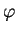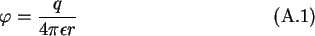Next: A.2 Self Capacitance of Up: A Capacitance Calculations Previous: A Capacitance Calculations

# A.1 Self Capacitance of a Sphere

The self-capacitance of a sphere is calculated from the potentialof a point charge q.This potential has spheres as equipotential surfaces, with the center of the spheres identical to the location of the point charge. Thus, if one inserts a conducting sphere with its center at the location of the point charge the field outside the sphere will not change, since the surface of the conductor coincides with an equipotential surface of the field. The self-capacitance is thereforewith d the diameter of the sphere.

Christoph Wasshuber